# Intermediate Rail 2MHz Switching Power Supply Withstands Entire Automotive Input Voltage Range

### Abstract

This application note illustrates an intermediate 8V switching power supply for an automotive radio and infotainment system. The design withstands the complete automotive input voltage range (including cold crank and load dump conditions), assuring a stable 8V supply for common subsystems such as a CD driver, LCDs, and a radio module in modern infotainment systems. To avoid disturbance in the AM and FM bands, the switching power supply runs at a fixed frequency of 2MHz, enabling an ideal solution for radio systems.

A similar version of this article appears on EDN, October 31, 2012.

### Introduction

Due to the increasing use of start-stop vehicle technology (which automatically turns off the engine while it is idling), more and more automotive systems must operate at low input voltages. These low input voltages occur in situations such as warm crank (when the battery voltage can drop as low as 6V) or cold crank (when the battery voltage can drop as low as 3V). Figure 1 illustrates common automotive systems that require different architecture solutions.
In some systems where the main power supply is 3.3V, a front-end buck converter with low dropout voltage may be sufficient (Case 1). If needed, a boost converter can operate off the 3.3V to regulate 5V (e.g., for the CAN transceiver) or other higher voltages (Case 2). Some systems operate with 5V or higher voltage rails, which require a front-end "preboost" to ensure that the input voltage to a buck converter never falls below a specific voltage (Case 3). This design pertains to the last case.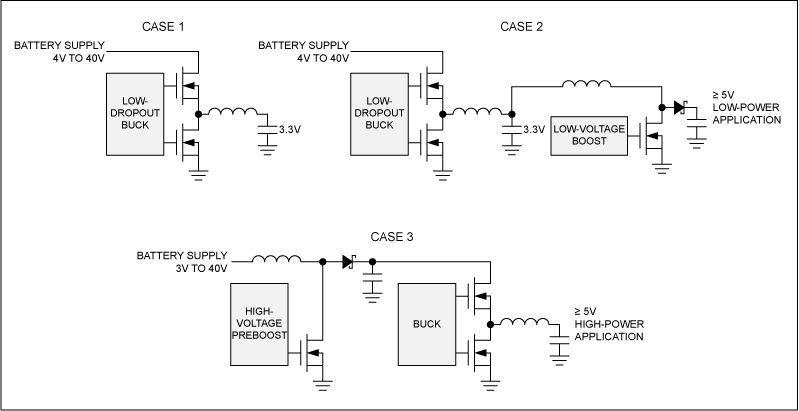Figure 1. Automotive power-supply solutions.
Low electromagnetic radiation is also a critical requirement in automotive systems, especially in the sensitive AM band. The design presented here meets this challenge by operating the switching converters above the AM band, i.e., at frequencies guaranteed to be above 1.71MHz (the high end of the MW band). Operating switching converters at high frequencies also reduces the size and cost of external passive components.
Figure 2 shows the switching power-supply schematic. It incorporates the MAX15005 step-up controller and the MAX16952 step-down controller, with additional circuitry for proper operation. Both ICs are synchronized with an external 2MHz square-wave logic signal, provided by a microcontroller or dedicated IC. This allows great flexibility in choosing the optimal switching frequency for the power supply. During normal battery conditions, the MAX15005 is disabled and the MAX16952 regulates the 8V on the OUTB node. When the battery voltage decreases during cold crank, the MAX15005 is enabled and boosts the voltage on the OUTA node. This allows the MAX16952 to regulate the 8V on the OUTB node. The entire design can survive up to 40V automotive load dump, thanks to the robustness of the two ICs. The system has been set up and tested to provide 20W of power (8V at 2.5A) on its main output (OUTB), although the external components can be modified to reach higher output power.Figure 2. The switching power-supply schematic.

### Output Voltage and Switching Frequency

To have 8V regulated on the OUTB node, it is necessary to properly choose the feedback resistor-divider (composed of the R22 and R21 resistors). Choosing a 51kΩ low-side resistor-divider for R22 (since the MAX16952 data sheet recommends low-side resistors of less than 100kΩ), the high-side resistor-divider must be selected using the following equation: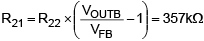(Eq. 1)
Where VFB = 1V (typ).
Selecting a standard resistor value of 360kΩ for R22, the typical resulting output voltage value is:(Eq. 2)
Assuming the resistors have a 1% tolerance, the minimum and maximum voltage values for the entire switching power supply (OUTB) are:(Eq. 3)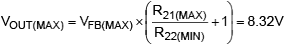(Eq. 4)
Where VFB(MIN) is 0.985V and VFB(MAX) is 1.015V.
As recommended in the data sheet, the external frequency must be higher than 110% of the MAX16952's internal selected frequency. Since we are synchronizing the MAX16952's switching frequency with an external 2MHz signal, we must choose an R16 internal oscillator resistance that imposes an internal switching frequency that is less than 1.8MHz. For this reason, a 30kΩ resistor was selected for R16. To make the MAX16952 switch at a 2MHz fixed frequency, it is necessary to avoid the dropout condition. The MAX16952 avoids dropout until the turn-off time (tOFF) is higher than 100ns (typ). This implies that the system can never go over a maximum duty cycle of:(Eq. 5)
Considering an efficiency (Eff) of 90% for the step-down regulator, the minimum input voltage for the MAX16952 (OUTA) that is able to ensure 2MHz fixed frequency switching is: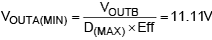(Eq. 6)
This means that the OUTA voltage must never be less than the 11.11V threshold. To guarantee an OUTA voltage that is always above 11.11V, the MAX15005 step-up controller must be enabled when the battery voltage (IN node) goes below 11.5V, leaving a margin of approximately 390mV for voltage drop across the L1 inductor and D2 Schottky diode.
During the 40V load dump peak, the OUTA voltage reaches its higher voltage value and the MAX16952 must regulate the 8V on its output. Thus, during load dump peak, MAX16952 should operate with a duty cycle of: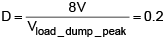(Eq. 7)
The MAX16952's minimum turn-on time (tON) is 80ns (typ), which enables it to reach a minimum duty cycle of: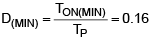(Eq. 8)
at a 2MHz switching frequency.
A minimum duty cycle of 0.16 ensures the 8V regulation during the 40V load dump.

### Inductor and Current SenseFigure 3. The MAX16952 inductor current.
Reducing the inductor peak current by using a large inductance value increases the efficiency of the step-down regulator; accomplishing this, however, requires a larger printed-circuit board (PCB) area and results in degradation of the load regulation. An acceptable compromise is to choose the inductor value in a manner to obtain a LIR (the ratio of the inductor peak-to-peak AC current to the DC average current) equal or lower than 0.3. Considering the following equations based on Figure 3:(Eq. 9)(Eq. 10)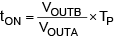(Eq. 11)
Merging these equations together, a single formula can indicate the L value:(Eq. 12)
Thus the minimum inductor value to reach a LIR factor equal or lower than 0.3 during a normal condition (OUTA = 12V) is: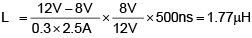(Eq. 13)
Using a standard inductor L2 of 2.2µH results in a LIR factor of 0.24 with a peak inductor current of:(Eq. 14)
The current limit is triggered when the voltage across the R20 sense resistor reaches 68mV (min). Leaving margin for the tolerance on the inductor, the sense resistor has been sized to have a voltage drop of 60% of the current limit threshold when the inductor current reaches its peak value (IPEAK):(Eq. 15)
A standard 15mΩ resistor for R20 has been chosen.

### UVLO Threshold

The first step in selecting external components for the MAX15005 step-up converter is determining the external undervoltage lockout (UVLO) threshold. This is done by selecting the resistor-divider that connects the main input IN pin, the ON/OFF pin, and ground. For this design we turned off the device when the input voltage was lower than 5V, assuming there would be a higher voltage during the cold-crank phase. Having selected a 100kΩ resistor for R5, the following equation is applied to choose the R4 resistor value:(Eq. 16)
A standard 300kΩ resistor was selected for R4.

### Overvoltage Input (OVI)

As discussed earlier for the MAX16952, we must guarantee that the voltage on the OUTA node is never lower than 11.11V to keep the MAX16952 out of dropout. Considering this voltage threshold and adding a reasonable voltage drop for the inductor L1 and the diode D2, the MAX15005 must turn on when the IN voltage goes below 11.5V. However, to optimize efficiency, the MAX15005 must not run when the battery voltage is at its normal level (IN = 12V).
To accomplish this, a resistor-divider connecting the IN pin, OVI pin, and ground is used to enable or disable the MAX15005, in accordance with the main supply value. The MAX15005 is disabled when the voltage at the OVI pin exceeds the 1.228V voltage threshold; it turns on again when the OVI pin voltage falls to 1.228V less a typical hysteresis of 125mV. Choosing a low-side R2 resistor-divider equal to 20kΩ and considering that the MAX15005 should turn off when the input voltage rises above 11.6V, the high-side R1 resistor-divider must be chosen according to the following formula:(Eq. 17)
With a standard 170kΩ R1 resistor, the MAX15005 is disabled when the main voltage rises above 11.67V, leaving a margin of 330mV from the normal 12V IN battery voltage. Once considering the hysteresis on the OVI comparator, we can estimate the falling voltage value on the main supply that enables the MAX15005: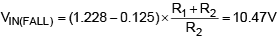(Eq. 18)
This result proves that the hysteresis is too large and we must reduce it to have a falling voltage threshold on the main supply of at least 11.5V. This can be done by adding a series resistance and Schottky diode (R3 and D1) between the OVI pin and SS pin. When the MAX15005 is disabled, the SS pin is internally tied to ground, connecting R3 in parallel with R2 and resulting in an effective decrease of the hysteresis. Using a 180kΩ resistor for R3 and neglecting the voltage drop across the diode, the new falling voltage threshold on the main supply becomes:(Eq. 19)
Using this configuration, it is possible to reach the target thresholds on both input voltage rising and falling edge. An alternative approach is to use an external comparator to monitor the main supply and drive the OVI input pin directly.

### Output VoltageFigure 4. The MAX15005 inductor current.
To maintain a 2MHz fixed switching frequency, it is necessary to respect the 170ns minimum tON, as stated in the MAX15005 data sheet, under all application conditions. The minimum tON results in a minimum duty cycle of 34% (with the 2MHz switching frequency), which limits the minimum output voltage that can be regulated with the MAX15005. To estimate this voltage threshold, it is necessary to consider the boost regulator duty cycle formula:(Eq. 20)
The minimum duty cycle is reached when the input voltage (VIN) is at its maximum value (11.67V in this design) and the MAX15005 is operating. It is possible to estimate the minimum output voltage that is regulated by the MAX15005 in this limit condition by adapting the previous equation:(Eq. 21)
The calculation has been performed in both minimum duty cycle and maximum input voltage conditions, considering a voltage drop across the D2 Schottky diode of 0.3V, and neglecting the voltage drop on the NMOS N1. Thus, the MAX15005 must regulate an output voltage greater than 17.38V to ensure the 2MHz switching frequency under all operating conditions.
Choosing a low-side feedback resistor-divider R13 equal to 10kΩ, it is possible to calculate the high-side feedback R14 resistor-divider: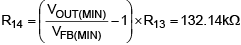(Eq. 22)
Where VFB(MIN) = 1.215V.
Finally, using a 1% tolerance 137kΩ resistor for R14, the minimum output voltage regulated by the operating MAX15005 is: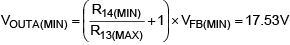(Eq. 23)
This ensures that the MAX15005 always switches at a 2MHz fixed frequency.
Assuming the output power of the design equals 20W (8V at 2.5A) and a 90% efficiency of the MAX16952, the MAX15005 output power must be at least 22.3W. Thus the average output current of the MAX15005 is 1.27A, considering a 17.53V regulated output voltage. Regulating higher output voltages with the MAX15005 reduces the output current, requiring a lower-cost D2 Schottky diode. However, the C7 output capacitor must be able to sustain the output voltage regulated by the MAX15005 itself.

### Synchronization and the Maximum Duty Cycle

To guarantee the external synchronization for the MAX15005 switching frequency, the frequency must be at least 102% higher than the programmed internal oscillator frequency. By choosing a 7kΩ resistor for R6 and a 100pF capacitor for C4, the MAX15005 internal oscillator frequency is approximately 1MHz, allowing an external synchronization frequency of 2MHz.
When the SYNC input detects a rising edge of the synchronization signal, the C4 capacitor is discharged through an internal 1.33mA current source (typ). When the voltage on this capacitor (RTCT pin) reaches 500mV, the C4 capacitor is charged through R6, which is connected to the VREG5 pin until the next synchronization signal rising edge is detected. The discharge time (TDISCHARGE) determines the minimum tOFF for the regulator. If this time is less than 160ns (as in this case), the minimum tOFF is clamped to 160ns. In fact assuming a charge time (TCHARGE) of 340ns (TP = 500ns), the voltage on RTCT increases:(Eq. 24)
Considering a 615µA¹ net discharging current during the discharge phase, the increased voltage on the RTCT pin is discharged in a time equal to:(Eq. 25)
A minimum tOFF of 160ns implies a maximum duty cycle of 68%. Reusing the boost regulator duty cycle formula (Equation 20) applied in the case where the maximum duty cycle is required (lower input voltage, in this case 5V), the maximum voltage regulated by the MAX15005 on the OUTA pin is: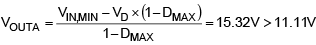(Eq. 26)
This voltage value guarantees MAX16952 operation outside of the dropout condition.

### Inductor Selection

A constraint in properly choosing the inductor value relates to the boost regulator's minimum output current. To ensure that regulator always operates in continuous mode, the minimum inductance value is:(Eq. 27)
In this design, the worst-case condition is when VIN is at its maximum value (11.67V) with a corresponding duty cycle of 37%.
With a 1A minimum current at the 8V stage and 90% efficiency of the buck converter, the boost regulator's minimum output power becomes 9.44W. This power corresponds to a 538mA minimum output current IOUTA(MIN), sourced from the boost regulator. Merging these last considerations and solving the previous equation, the minimum inductance value is 1.32µH. For this design, a 2.2µH inductor was chosen for L1.

### Current Sense

The MAX15005 current limit is triggered when the voltage across the sense resistor reaches the typical value of 305mV. Consequently, to properly select this resistor it is necessary to calculate the peak current in the boost inductor: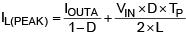(Eq. 28)
The peak value is reached when the input voltage is at its minimum; in this case, it is 5V and the maximum duty cycle is 68%. As previously calculated, the boost output voltage (OUTA pin) is 15.23V, requiring an IOUTA current of 1.46A to provide the necessary power to MAX16952. In a worst-case scenario, the inductor peak current is 4.95A. An appropriate margin is to design the sense resistor to have a 200mV voltage drop when the inductor current is at its peak:(Eq. 29)
Thus a 40MΩ resistor was chosen for R10.

### Cold-Crank Test

A cold-crank test was performed on the bench, forcing the main supply voltage (IN) to decrease from 12V to 7V within 10ms. As Plot 1 shows, when the IN voltage decreases, the MAX15005 begins pumping OUTA voltage up to 17.5V. This allows the MAX16952 to keep 8V regulated on OUTB. On the other side, when the input voltage returns to its operating value, the MAX15005 stops and the OUTA voltage decreases to the IN voltage, less the drop on the D2 diode and L1 inductor. Every test has been performed with an 2.5A output load on the OUTB pin.

### Plot 1Plot 2 and Plot 3 illustrate a closer view of the cold-crank fall and rise phases, respectively.

### Plot 2### Plot 3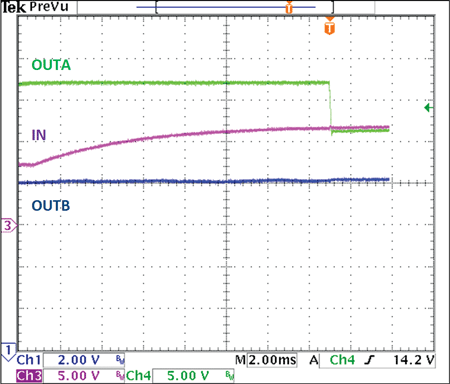### Analyzing the Frequency Domain

With the help of an oscilloscope's embedded FFT tool, the frequency spectrum of the voltage on the MAX16952 switching node LX_Buck pin during cold crank is shown in Plot 4 (IN voltage fall) and 5 (IN voltage rise). Notice that the frequency spectrum contains the 2MHz frequency, the related harmonics, and, of course, the DC component. There is no AC component below 2MHz, preventing noise disturbance in the AM band.

### Plot 4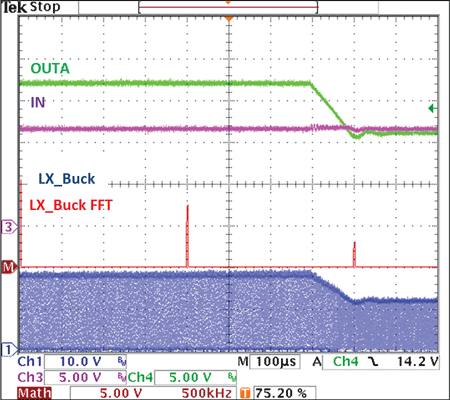### Plot 5The same procedure has been performed for the MAX15005 switching node LX_Boost, highlighting the 2MHz frequency, harmonics, DC component, and eliminating AM band noise.

### Plot 6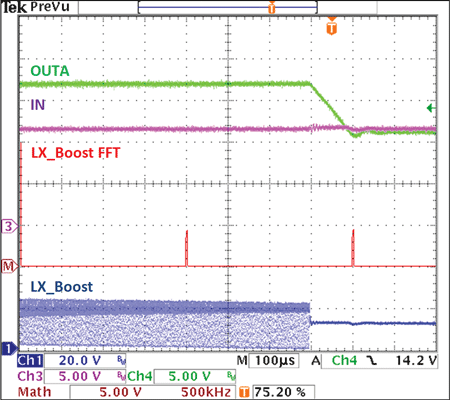### Plot 7### Further Improvements

To optimize efficiency, designers can bypass the D2 Schottky diode during the normal application condition when the MAX15005 is nonoperational. This is accomplished with an N-channel MOSFET connected in parallel with D2 when the main supply is at its normal value. To reduce electromagnetic interference (EMI), slow the voltage edge on the MOSFET gate and add external resistors (R8, R17, R18, and R19), resulting in a trade-off of increased power dissipation. To filter out spikes on the current-sense waveform of the MAX15005, it can be useful to introduce a small RC filter (C6 and R9). The MAX15005 current limit threshold can also be reduced by adding an offset to the R7 resistor—this reduces power dissipation across the sense resistor R10.

#### References

1. This estimate assumes an internal 1.33mA discharging current and an external charging current sourced from VREG5 through R6 as VREG/R6 = 715µA. The latter estimation approximates the voltage on RTCT pin as 0V.